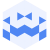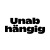Am 27. Sep. 2022 | 19:45 Uhr

# Sept 27 2022 | Tresor Linz | canceled but not canceled I Emilie Škrijelj and Krach der RoboterCreated at 26. Sep. 2022

144 Ansichten
##### by Tresor Linz

<<<<<<<<<<<<<<<<<<<<<<<<<<<<<<<<<<<<<<<<<<<<<<<<<<<<<<<<<
GLITCH - Emilie Škrijelj
<<<<<<<<<<<<<<<<<<<<<<<<<<<<<<<<<<<<<<<<<<<<<<<<<<<<<<<<<
Emilie Škrijelj explores the accordion in its smallest folds and uses it both as a percussion instrument and a generator of electroacoustic materials. Inspired by her research around the turntable, modular synths and field recording, she brings the accordion to the abstract territory of electronics by manipulating the bellows and exploring its extremities.
In the solo Glitch, she extends the accordion using salvaged objects; an accordion prepared in three dimensions.

In 2020, she created with Tom Malmendier the label eux sæm dedicated to improvised and experimental music which brings together some of her current projects such as the quartet "nuits" or the duo "Les Marquises" and develops the Presque île project, a series of small sound forms in situ, created for the landscape and which they group together on a sound map.

<<<<<<<<<<<<<<<<<<<<<<<<<<<<<<<<<<<<<<<<<<<<<<<<<<<<<<<<<
Krach der Roboter
<<<<<<<<<<<<<<<<<<<<<<<<<<<<<<<<<<<<<<<<<<<<<<<<<<<<<<<<<

Krach der Roboter ist als infektionsrisikofreie künstliche Intelligenz perfekt geeignet für Live-Sound Performances. Seine reflektierende aseptische Metalloberfläche ist optimiert für Streamingeinsätze. Nicht nur klanglich sondern auch quasi ganzheitlich stellt Krach eine Ausnahmeerscheinung dar, die zukunftsweisend für ein friedliches und gleichberechtigtes Miteinander von Mensch und Maschine steht.

Musikalisch erstreckt sich Krachs Algorithmenhorizont von redundant-meditativem Geblubbere über poppige Volksweisen bis zu energetisch aufgeladenem Metallgewitter hin. Gelegentlich auch all dies gleichzeitig.

<<<<<<<<<<<<<<<<<<<<<<<<<<<<<<<<<<<<<<<<<<<<<<<<<<<<<<<<<
Tresor Linz
<<<<<<<<<<<<<<<<<<<<<<<<<<<<<<<<<<<<<<<<<<<<<<<<<<<<<<<<<

Raum für Klangkunst und künstlerische Experimente.

Space for sound art and artistic experiments.

##### Share & Embed
Embed this Video